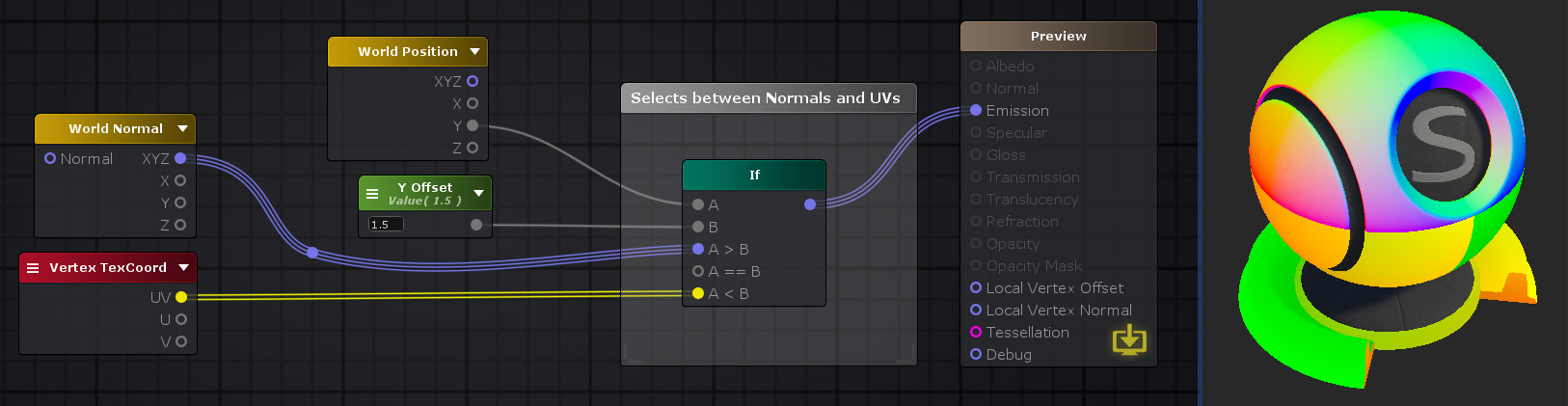## If Node

The If node compares two float inputs A and B and outputs a value from on one of its inputs A > B , A == B or A < B according to the comparison result.Nodes used: World Position, Float, World Normal, Vertex TexCoord, If

Node Parameter Description Default Value
A First value of the comparison operation. Only visible if the respective input port is not connected. 0
B Second value of the comparison operation. Only visible if the respective input port is not connected. 0
Dynamic Branching Toggle to let the compiler know this conditional operation should be compiled on an actual branch. False

Input Port Description Type
A First value of the comparison operation. Float
B Second value of the comparison operation. Float
A > B Value to be outputted if value of A is greater than value of B. Float 
A == B Value to be outputted if value of A is equal to value of B. Float 
A < B Value to be outputted if value of A is less than value of B. Float 

1. ^ Port automatically adapts to all connection types except Sampler types.